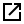# CreateHotSpotGrid Method

## Description

Creates a Hot Spot Grid item from the origins of the Items in a Named List## Syntax

Visual Basic
```Public Function CreateHotSpotGrid( _
ByVal list As String, _
ByVal formula As String, _
ByVal kernel As Long, _
ByVal type As Long, _
ByVal unit2 As Long, _
ByVal coincident As Long, _
ByVal bandwidth As Double, _
ByVal ox As Double, _
ByVal oy As Double, _
ByVal oz As Double, _
ByVal cx As Double, _
ByVal cy As Double, _
ByVal flags As Long _
) As Integer```

## Parameters

list
A Named List which contains the Items from which to make a scatter Grid.

The origin of each Item is used to seed a value in the Grid item. The Items will typically be PointItems but any Item class may be used. The Items are not edited by this operation and therefore can be from a read-only dataset.

formula

An expression which is evaluated for each item in the Named List.

kernel

This can be one from the following list:

 `SIS_KERNEL_DENSITY_FUNCTION_NORMAL  ` Normal SIS_KERNEL_DENSITY_FUNCTION_QUARTIC Quartic SIS_KERNEL_DENSITY_FUNCTION_NEG_EXP Negative Exponential SIS_KERNEL_DENSITY_FUNCTION_NEG_EXP_BOUNDED Negative Exponential (bounded) SIS_KERNEL_DENSITY_FUNCTION_TRIANGULAR Triangular (Conic) SIS_KERNEL_DENSITY_FUNCTION_UNIFORM Uniform (Constant) SIS_KERNEL_DENSITY_FUNCTION_EPANECHNIKOV Epanechnikov (Quadratic) SIS_KERNEL_DENSITY_FUNCTION_TRIWEIGHT Triweight (tricube) SIS_KERNEL_DENSITY_FUNCTION_NEG_COSINE Cosine

The above kernels are described in the Kernel Density Estimations (KDE) section of the Spatial Analysis topic.

type
 `SIS_KERNEL_DENSITY_GRID_TYPE_PROBABILITY  ` All grid cells add up to 1.0. Each grid cell value is the probability of an event happening at this cell. SIS_KERNEL_DENSITY_GRID_TYPE_REL_DENSITY 2D unit needs to be provided, i.e. m^2, miles^2, km^2, etc. Each grid cell value is the number of events per m^2, miles^2, km^2, etc. SIS_KERNEL_DENSITY_GRID_TYPE_ABS_DENSITY All grid cells add up to N, where N is the number of input points. Each grid cell value is the number of events per grid cell area.
unit2
This is the 2-D unit to be used for relative density grids. Use the 2D unit constants provided: SIS_UNIT2_M, etc. See SetCoordUnits Method.

coincident
 `SIS_KERNEL_DENSITY_COINCIDENT_POINTS_SUM  ` Uses the total of coincident point values. SIS_KERNEL_DENSITY_COINCIDENT_POINTS_AVERAGE Uses the average of the coincident points. SIS_KERNEL_DENSITY_COINCIDENT_POINTS_MIN Uses the minimum value of the coincident points. SIS_KERNEL_DENSITY_COINCIDENT_POINTS_MAX Uses the maximum value of the coincident points.

Note: When all incidents have identical weight it makes no difference whether you select coincident points to be min, average, or max. The points only have an effect if incidents can have different weights, i.e. once an expression has been specified.

bandwidth

This is the bandwidth to use for creating the grid.

ox
The x coordinate of the origin of the grid to be created.

oy
The y coordinate of the origin of the grid to be created.

oz
The z coordinate of the origin of the grid to be created.

cx
The x size of each grid cell in meters.

cy

The y size of each grid cell in metres.

flags

1 - grid uses no-data values.

0 - grid does not use no-data values.

## Remarks

Available: GEO OD SISpy

Groups:

## Example

sis.CreateHotSpotGrid ("NamedItemList", "", sis.SIS_KERNEL_DENSITY_FUNCTION_EPANECHNIKOV, sis.SIS_KERNEL_DENSITY_GRID_TYPE_PROBABILITY, sis.SIS_UNIT2_M, sis.SIS_KERNEL_DENSITY_COINCIDENT_POINTS_SUM, 500, 0, 0, 0, 250, 250, 1)